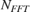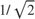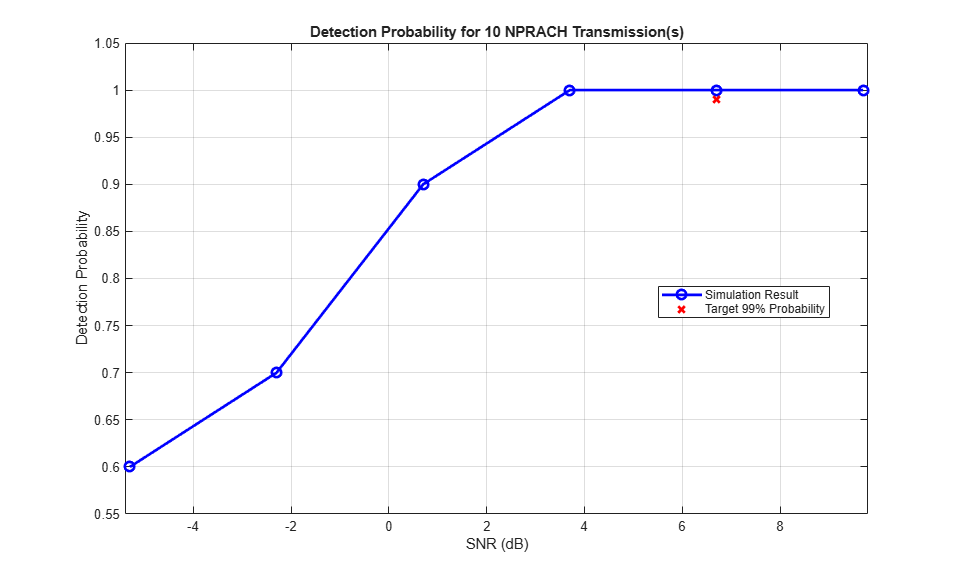# NB-IoT PRACH Detection and False Alarm Conformance Test

This example implements the narrowband physical random access channel (NPRACH) missed detection and false alarm conformance tests for frame structure type 1, as defined in TS 36.141. You can measure the probability of correct detection of the NPRACH preamble in the presence of a preamble signal or switch the NPRACH transmission off to measure the false alarm probability.

### Introduction

The NPRACH is a narrowband internet of things (NB-IoT) uplink transmission used by the user equipment (UE) to initiate synchronization with the eNodeB. Section 8.5.3 of TS 36.141 defines that the probability of NPRACH detection must be greater than or equal to 99% at the SNR levels listed in Table 8.5.3.5-1 for several combinations of preamble format, number of repetitions, and channel propagation conditions. There are several detection error cases:

• Detecting an incorrect preamble

• Not detecting a preamble

• Detecting the correct preamble but with the wrong timing estimation

TS 36.141 states that a correct detection is achieved when the estimation error of the timing offset of the strongest path is less than 3.646 microseconds.

In this example, an NPRACH waveform is configured and passed through an appropriate channel. At the receiver side, the example performs NPRACH detection and calculates the NPRACH detection probability. The example considers the parameters defined in Table 8.5.3.1-1 and Table 8.5.3.5-1 of TS 36.141. These are: frequency-division duplexing (FDD), 2 receive antennas, 8 repetitions, EPA1 channel, preamble format 0, SNR 6.7 dB. If you change the simulation scenario to use one of the other NPRACH preamble formats, propagation channel, or number of repetitions listed in Table 8.5.3.5-1, you need to update the NPRACH configuration according to Table 8.5.3.1-1 and you need to update the values of the frequency offset and the SNR according to Table 8.5.3.5-1.

### Simulation Configuration

The example considers 10 NPRACH transmissions at a number of SNRs. You should use a large number of numNPRACHTransmissions to produce meaningful results. You can set SNRdB as an array of values or a scalar. Table 8.5.3.5-1 of TS 36.141 specifies the frequency offset foffset that is modeled between the transmitter and receiver. The timeErrorTolerance variable specifies the time error tolerance, as defined in Section 8.5.3.1 of TS 36.141. You can set the detection threshold to a value in the range [0,1] or to empty to use the default value in the hNPRACHDetect function. To simulate a false alarm test, disable the NPRACH transmission by setting nprachEnabled to false instead.

numNPRACHTransmissions = 10; % Number of NPRACH transmissions to simulate at each SNR SNRdB = [-5.3 -2.3 0.7 3.7 6.7 9.7]; % SNR range in dB foffset = 200.0; % Frequency offset in Hz timeErrorTolerance = 3.646; % Time error tolerance in microseconds threshold = []; % Detection threshold nprachEnabled = true; % Enable NPRACH transmission. To simulate false alarm test, disable NPRACH transmission. 

### UE Configuration

UE settings are specified in the structure ue.

ue.NBULSubcarrierSpacing = '15kHz'; % Uplink subcarrier spacing ('3.75kHz', '15kHz') ue.NNCellID = 0; % Narrowband cell identity ue.Windowing = 0; % Windowing samples 

### NPRACH Configuration

Table 8.5.3.1-1 of TS 36.141 specifies the NPRACH configurations to use for the NPRACH detection conformance test.

Set the NPRACH configuration structure nprach and compute the NPRACH resource information nprachInfo by calling lteNPRACHInfo.

nprach = struct(); nprach.NPRACHFormat = '0'; % Preamble format ('0','1','2') nprach.Periodicity = 80; % Resource periodicity in ms nprach.SubcarrierOffset = 0; % Frequency location of the first allocated subcarrier nprach.NumSubcarriers = 12; % Number of allocated subcarriers nprach.NRep = 8; % Number of repetitions nprach.StartTime = 8; % Starting time in ms nprach.NInit = 0; % Initial subcarrier nprach.NPRACHPower = 0; % Power scaling in dB nprachInfo = lteNPRACHInfo(ue,nprach); % NPRACH resource information 

### Propagation Channel Configuration

Configure the propagation channel model using the chcfg structure as described in Table 8.5.3.5-1 of TS 36.141.

chcfg.NRxAnts = 2; % Number of receive antennas chcfg.DelayProfile = 'EPA'; % Delay profile chcfg.DopplerFreq = 1; % Doppler frequency chcfg.MIMOCorrelation = 'Low'; % MIMO correlation chcfg.Seed = 42; % Channel seed. Change this for different channel realizations chcfg.NTerms = 16; % Oscillators used in fading model chcfg.ModelType = 'GMEDS'; % Rayleigh fading model type chcfg.InitPhase = 'Random'; % Random initial phases chcfg.NormalizePathGains = 'On'; % Normalize delay profile power chcfg.NormalizeTxAnts = 'On'; % Normalize for transmit antennas chcfg.SamplingRate = nprachInfo.SamplingRate; % Sampling rate % Compute the maximum channel delay chcfg.InitTime = 0; [~,chInfo] = lteFadingChannel(chcfg,0); % Get channel info maxChDelay = ceil(max(chInfo.PathSampleDelays)) + chInfo.ChannelFilterDelay; 

### Loop for SNR Values

Use a loop to run the simulation for the set of SNR points given by the vector SNRdB. The SNR vector configured here is a range of SNR points including a point at 6.7 dB, the SNR at which the test requirement for NPRACH detection rate (99%) is to be achieved for preamble format 0, as discussed in Table 8.5.3.5-1 of TS 36.141.

lteNPRACH generates an output signal normalized to the same transmit power as for an uplink data transmission within the LTE Toolbox™. Therefore, the same normalization must take place on the noise added to the NPRACH. The noise added before OFDM demodulation will be amplified by the IFFT by a factor equal to the square root of the size of the IFFT (). To ensure that the power of the noise added is normalized after demodulation, and thus to achieve the desired SNR, the desired noise power is divided by. In addition, as real and imaginary parts of the noise are created separately before being combined into complex additive white Gaussian noise, the noise amplitude is scaled byso the generated noise power is 1.

At each SNR test point, calculate the probability of detection for each NPRACH transmission using these steps:

• NPRACH Transmission: Use lteNPRACH to generate an NPRACH waveform. Send the NPRACH preambles with a fixed timing offset of 50% of the number of cyclic shifts, as defined in Section 8.5.3.4.2 of TS 36.141.

• Noisy Channel Modeling: Pass the waveform through a fading channel and add additive white Gaussian noise. Add additional samples to the end of the waveform to cover the range of delays expected from the channel modeling (a combination of implementation delay and channel delay spread). This implementation delay is then removed to ensure the implementation delay is not interpreted as an actual timing offset in the preamble detector.

• Application of Frequency Offset: Apply the frequency offset to the received waveform as defined in Table 8.5.3.5-1 of TS 36.141.

• NPRACH Detection: Perform NPRACH detection using the hNPRACHDetect function for all initial subcarrier indices NInit, each one of which uniquely defines an NPRACH preamble. Use the detected NPRACH index and offset returned by hNPRACHDetect to determine whether a detection was successful according to the constraints discussed in the Introduction section.

% Initialize variables storing probability of detection at each SNR pDetection = zeros(size(SNRdB)); % The temporary variables 'chcfg_init' and 'chInfo_init' are used to create % the temporary variables 'chcfg' and 'chInfo' within the SNR loop to % create independent instances in case of parallel simulation chcfg_init = chcfg; chInfo_init = chInfo; for snrIdx = 1:length(SNRdB) % comment out for parallel computing % parfor snrIdx = 1:numel(SNRdB) % uncomment for parallel computing % To reduce the total simulation time, you can execute this loop in % parallel by using the Parallel Computing Toolbox(TM). Comment out the % 'for' statement and uncomment the 'parfor' statement. If the Parallel % Computing Toolbox is not installed, 'parfor' defaults to normal 'for' % statement % Display progress in the command window timeNow = char(datetime('now','Format','HH:mm:ss')); fprintf([timeNow,': Simulating SNR = %+5.1f dB...'],SNRdB(snrIdx)); % Initialize the random number generator stream rng('default'); % Initialize variables for this SNR point, required for initialization % of variables when using the Parallel Computing Toolbox chcfg = chcfg_init; chInfo = chInfo_init; % Normalize noise power to account for the sampling rate, which is a % function of the IFFT size used in OFDM modulation. The SNR is defined % per carrier resource element for each receive antenna. ueInfo = lteSCFDMAInfo(ue); SNR = 10^(SNRdB(snrIdx)/10); N0 = 1/sqrt(2.0*double(ueInfo.Nfft)*SNR); % Detected preamble count detectedCount = 0; % Loop for each NPRACH transmission for nprachIdx = 1:numNPRACHTransmissions % Generate NPRACH waveform waveform = generateWaveform(ue,nprach,nprachInfo,nprachEnabled); % Set NPRACH timing offset in microseconds as per Section 8.5.3.4.2 of TS 36.141 timingOffset = 0.5*nprachInfo.PreambleParameters.T_CP/30720*1e3; % (microseconds) sampleDelay = fix(timingOffset/1e6*nprachInfo.SamplingRate); % Generate transmit waveform txwave = [zeros(sampleDelay,1); waveform]; % Pass data through channel model rxwave = applyChannel(txwave,chcfg,nprachInfo.Nfft,nprach.Periodicity,nprachIdx,maxChDelay); % Add noise noise = N0*complex(randn(size(rxwave)),randn(size(rxwave))); rxwave = rxwave + noise; % Apply frequency offset t = ((0:size(rxwave,1) - 1)/chcfg.SamplingRate).'; rxwave = rxwave.*repmat(exp(1i*2*pi*foffset*t),1,size(rxwave,2)); % NPRACH detection for all frequency hopping patterns [detected,offset,detinfo] = hNPRACHDetect(ue,nprach,rxwave,threshold); % Test for frequency hopping pattern detection if (length(detected)==1) if ~nprachEnabled % For the false alarm test, any preamble detected is wrong detectedCount = detectedCount + 1; else % Test for correct frequency hopping pattern detection if (detected==nprach.NInit) % Calculate timing estimation error trueOffset = timingOffset/1e6; % (s) measuredOffset = offset/nprachInfo.SamplingRate; timingerror = abs(measuredOffset - trueOffset); % Test for acceptable timing error if (timingerror<=(timeErrorTolerance/1e6)) detectedCount = detectedCount + 1; % Detected preamble end end end end end % of NPRACH transmission loop % Compute final detection probability for this SNR pDetection(snrIdx) = detectedCount/numNPRACHTransmissions; % Display the detection probability for this SNR fprintf('Detection probability: %4.2f%%\n',pDetection(snrIdx)*100); end % of SNR loop 
12:11:38: Simulating SNR = -5.3 dB...

### Analysis

At the end of the SNR loop, the example plots the calculated detection probabilities for each SNR value against the target probability.

targetSNR = 6.7; NPRACHDetectionResults(pDetection,SNRdB,targetSNR,numNPRACHTransmissions,nprachEnabled);### References

1. 3GPP TS 36.141. "Base Station (BS) conformance testing." 3rd Generation Partnership Project; Technical Specification Group Radio Access Network; Evolved Universal Terrestrial Radio Access (E-UTRA).

2. 3GPP TS 36.211. "Physical channels and modulation." 3rd Generation Partnership Project; Technical Specification Group Radio Access Network; Evolved Universal Terrestrial Radio Access (E-UTRA).

### Local Functions

function waveform = generateWaveform(ue,nprach,nprachInfo,nprachEnabled) % Generate the waveform. If the NPRACH transmission is disabled, the % function generates a waveform of zeros. if nprachEnabled waveform = lteNPRACH(ue,nprach); else waveform = complex(zeros(nprach.Periodicity*nprachInfo.SamplingRate/1e3,1)); end end function rxwave = applyChannel(txwave,chcfg,nFFT,periodicity,nprachIdx,maxChDelay) % Pass data through channel model % Resample the transmit waveform to speed up the channel nFFT_mod = nFFT/8; txwave = resample(txwave,nFFT_mod,nFFT); chcfg.SamplingRate = chcfg.SamplingRate*nFFT_mod/nFFT; % Pass data through channel model. Append zeros at the end of the % transmitted waveform to flush channel content. These zeros take into % account any delay introduced in the channel. This is a mix of % multipath delay and implementation delay. This value may change % depending on the sampling rate and delay profile. chcfg.InitTime = (nprachIdx - 1)*periodicity/1000; [rxwave,chInfo] = lteFadingChannel(chcfg,[txwave; zeros(ceil(maxChDelay*nFFT_mod/nFFT),1)]); % Remove the implementation delay of the channel filter rxwave = rxwave((chInfo.ChannelFilterDelay + 1):end,:); % Resample the receive waveform back to the original sampling rate rxwave = resample(rxwave,nFFT,nFFT_mod); end function NPRACHDetectionResults(pDetection,SNRdB,targetSNR,numNPRACHTransmissions,nprachEnabled) % Plot detection probability figure('NumberTitle','off','Name','NPRACH Detection Probability'); plot(SNRdB,pDetection,'b-o','LineWidth',2,'MarkerSize',7); title(['Detection Probability for ',num2str(numNPRACHTransmissions),' NPRACH Transmission(s)']); xlabel('SNR (dB)'); ylabel('Detection Probability'); grid on; hold on; % Plot target probability if nprachEnabled % For a missed detection test, detection probability should be >= 99% pTarget = 99; else % For a false alarm test, detection probability should be < 0.1% pTarget = 0.1; end plot(targetSNR,pTarget/100,'rx','LineWidth',2,'MarkerSize',7); legend('Simulation Result', ['Target ',num2str(pTarget),'% Probability'],'Location','best'); minP = 0; if(~isnan(min(pDetection))) minP = min([pDetection(:); pTarget]); end axis([SNRdB(1)-0.1, SNRdB(end)+0.1, minP-0.05, 1.05]); end 
Detection probability: 60.00% 12:11:41: Simulating SNR = -2.3 dB...Detection probability: 80.00% 12:11:43: Simulating SNR = +0.7 dB...Detection probability: 100.00% 12:11:44: Simulating SNR = +3.7 dB...Detection probability: 100.00% 12:11:47: Simulating SNR = +6.7 dB...Detection probability: 100.00% 12:11:48: Simulating SNR = +9.7 dB...Detection probability: 100.00%